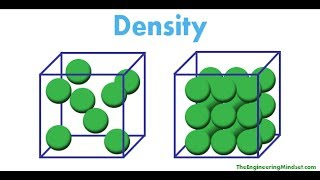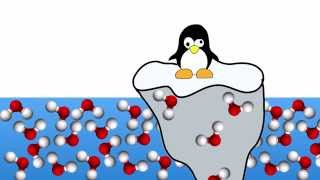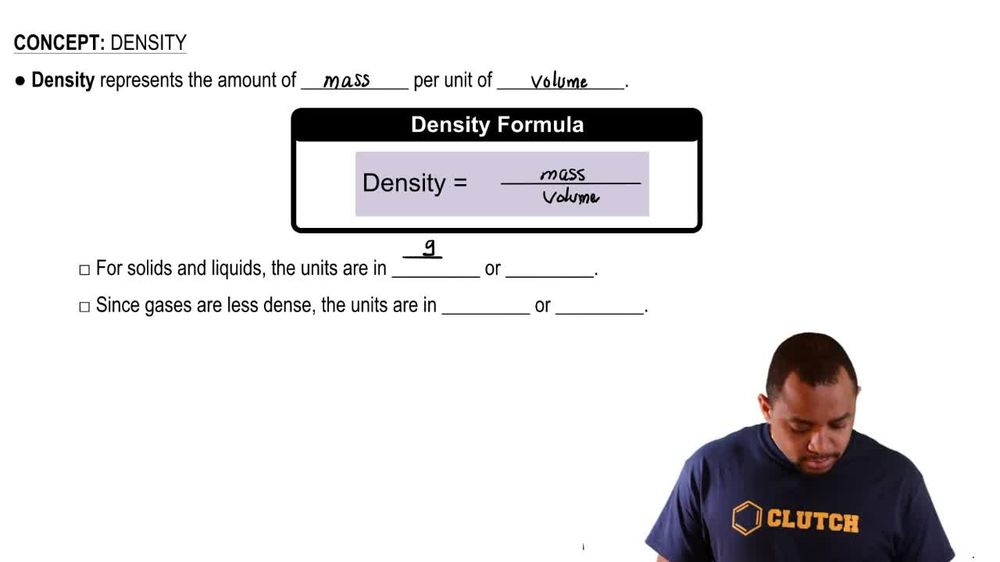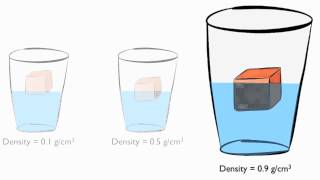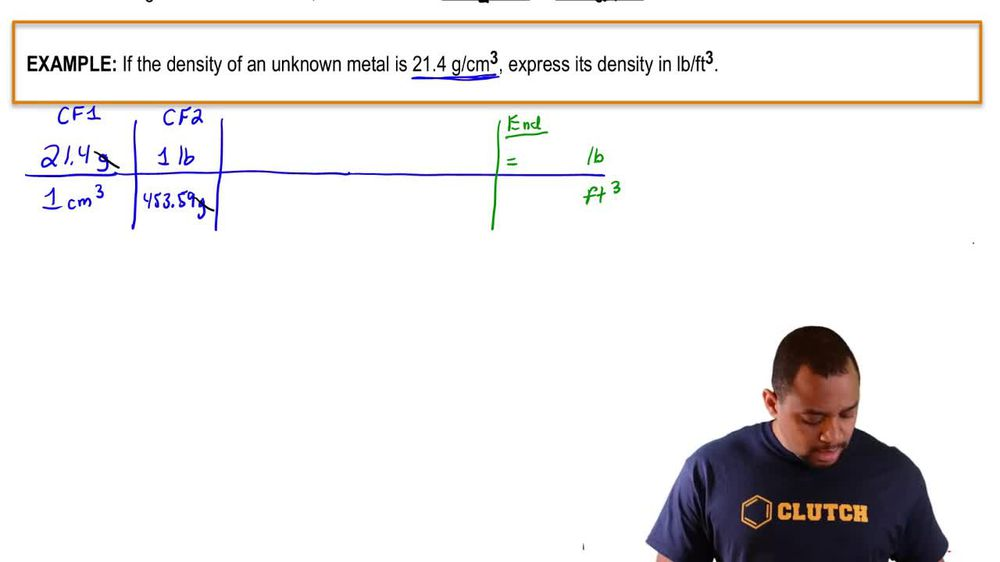Start typing, then use the up and down arrows to select an option from the list.
1. 1. Intro to General Chemistry2. Density
Problem

# Answer the following questions. (d) A particular sample of crude oil has a density of 0.85 g/mL. What is the mass of 1.00 barrel of this crude oil in kg, where a barrel of oil is exactly 42 gallons?

Relevant Solution1m
Play a video:
Hello everyone today. We have the following problem. The density of a sample of wine is 1.8 g per milliliter. If a salman Azar of wine is nine liters, what is the weight of 3.67 Salman Azar of wine in ounces. So first let's make note of a couple of conversions. One Simon Azar, As you go to nine L One leader is you go to 1000 middle leaders And of course one ounce Go to 28.35 g. So now we can set up our problem. We're gonna start with what we were given, which was 3.67 salman as are all agree that that is just sell. We're going to multiply that by the conversion factor, that one Sau man Azar is equal to nine liters. We will then convert those leaders into regular leader milliliters by saying that one leader is equal to 1000 militia leaders in the question stem. We have the density. So we're gonna use that 1.8 g per one millimeter. And then lastly, we're going to take the conversion factor that one ounce is equal to 28.35 g. And so now we have our units canceling out, we're left with ounces. 1.26 times 10 to the 3rd ounce is our final answer. I hope this helped. And until next time